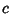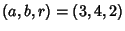## Spiric SectionA curve with Cartesian equationAround 150 BC,Menaechmus constructed Conic Sections by cutting a Cone by a Plane. Two hundred years later, the Greek mathematician Perseus investigated the curves obtained by cutting a Torus by a Plane which is Parallel to the line through the center of the Hole of the Torus (MacTutor).

In the Formula of the curve given above, the Torus is formed from a Circle of Radiuswhose center is rotated along a Circle of Radius. The value ofgives the distance of the cutting Plane from the center of the Torus.

When, the curve consists of two Circles of Radiuswhose centers are atand. If, the curve consists of one point (the origin), while if, no point lies on the curve. The above curves have, (3, 1, 2) (3, 0.8, 2), (3, 1, 4), (3, 1, 4.5), and (3, 0, 4.5).

References

MacTutor History of Mathematics Archive. Spiric Sections.'' http://www-groups.dcs.st-and.ac.uk/~history/Curves/Spiric.html.